Updated by lalmenda on Apr 30, 2020
REPORT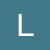lalmenda
Owner
12 items   1 followers   0 votes   1 views

1

## 5th Grade Math - Fifth Grade Math Games and Fun WorksheetsLearn and practice fifth grade math online for free. Check ✓ 5th Grade Math Games and ✓ Fun Math Worksheets ✓ Full Curriculum ✓ Fun Learning.

2

## math is fun!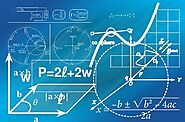4

## Key Math Skills Learned in 5th Grade

• How to divide whole numbers with and without remainders.
• How to make connections between decimals, fractions, and percentages.
• How to multiply and divide fractions.
• How to compute the area and volume of basic geometric shapes like rectangles and squares.
5

## Grade 5 - Practice with Math Games5th Grade Math - Fluency with fraction addition and subtraction, improve skill with fraction multiplication and division, decimal operations, developing understanding of volume.

6

## Edupress Mathological Liar Game, Grade 5 (EP63398)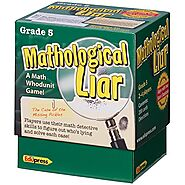Includes 50 math mysteries,Set of 200, 4" x 2.5" cards & directions,For 2-4 players

7

## 5th Grade Math | Free, Online Math Games | Math Playground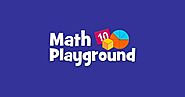8

## a 5th grade student on his way to learn about math!9

## Real-World Math, Grades 5-8 from Teacher Created Resources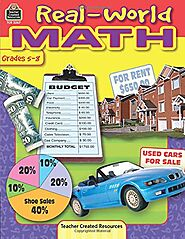Students learn to formulate solutions to real-life problems,Book includes guided practice and activity pages,A great way to work on algebra and teach real-world skills,Ideal for test prep,Works great for home or classroom use

10

## Fifth Grade Math | Education.com

11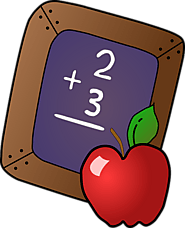1.Highlight Real-World Uses of Math.
2.Help Prepare for Math Class.
4.Keep Math Positive.
5.Consult Online Resources.
7.Practice Calculations Using Decimals.
8.Practice Using Fractions.

12

## IXL | Learn 5th grade math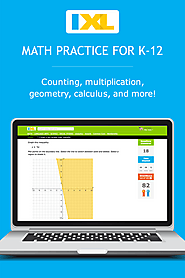Set students up for success in 5th grade and beyond! Explore the entire 5th grade math curriculum: multiplication, division, fractions, and more. Try it free!

13